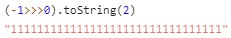In Cocos Creator, some physics components (there are currently rigid body components and collider components) provide interfaces for Groups and Masks.

## How does it work?

Physics elements and nodes are currently in a one-to-one relationship. The group and mask belong to the physics elements. The physics components on a single node modify the group and mask of the physics elements corresponding to the nodes.

As long as the following conditions are true, it will be detected

``````(GroupA & MaskB) && (GroupB & MaskA)
``````

For example: two physical elements `A` and `B`.

The group value of `A` is `1` and the mask value is `3`

The group value of `B` is `2`, and the mask value is `2`

The formula `(1 & 2) && (2 & 3)` is false, so here `A` will not be detected with `B`.

Here according to the mask value of `B` is `2`, we can know that the detectable group of `B` is `1`, and the group of `A` is `0`, so it is not detected.

Note: the expression depends on bit operation, the bit operation of JavaScript is limited to `32` bits, and the last bit is the sign bit. To avoid exceeding the operation range, it is recommended that the range of the group is `[0, 31 )`.

## Groups

### Setting a Group Value

The following group value is `3`, and the binary value is `11`, which means it is in the `0`, `1` group (starting from `0`)

``````const group = (1 << 0) + (1 << 1);
Collider.setGroup(group);
``````

### Obtaining a Group Value

Use `getGroup()`.

``````Collider.getGroup();
``````

Based on the above code, after the following code, the grouping value is `7`, and the binary value is `111`, so it means that it is in the `0`, `1`, and `2` groups.

``````const group = 1 << 2;
``````

### Removing a Group

Based on the above code, after the following code, the grouping value is `3`, so in the `0`, `1` group.

``````const group = 1 << 2;
Collider.removeGroup(group);
``````

Note: it is recommended to fix in a group.

Note: the receiving parameters of the above methods are all decimal numbers. For easy understanding, binary explanation is used here. Developers can also directly input decimal numbers for group operation after they are familiar**.

The value of the following mask is `3`, the binary value is `11`, indicating that the detectable group is `0`, `1`.

``````const mask = (1 << 0) + (1 << 1);
``````

Use `getMask()`

``````console.log(Collider.getMask());
``````

On the basis of the above code, after the following code, a detectable group `3` was added.

``````const mask = 1 << 2;
``````

The following code removes a detectable group `3`.

``````const mask = 1 << 2;
``````

Note: the addition and subtraction operation have higher priority than the shift operation. Note: flexible use of group and mask can reduce the cost of additional detecting.

## Examples

Here is a simple example of usage:

### Defining a Group

Method 1: Defined in an object

``````export const PHY_GROUP = {
Group0: 1 << 0, // Group 0 is equivalent to giving it an alias of Group0.
Group1: 1 << 1
};
``````

Method 2: Defined in an enum (TypeScript only)

``````enum PHY_GROUP {
Group0 = 1 << 0,
Group1 = 1 << 1
};
``````

In order to be able to set up groups on the panel, you need to register the defined groups to the editor `Enum(PHY_GROUP)` through the Enum function exported by the cc module.

Note: for historical reasons, the Enum function has special treatment for `-1`. If you are not familiar with it, do not define a property with a value of `-1`.

The mask can be defined according to grouping, for example:

• Define a mask(`const maskForGroup1 = PHY_GROUP.Group1;`) that only detects `Group1`
• Define a mask(`const maskForGroup01 = PHY_GROUP.Group0 + PHY_GROUP.Group1;`) that can detect `Group0` and `Group1`
• Define a mask(`const maskForNone = 0;`) that is not detected by all groups
• Define a mask(`const maskForAll = -1;`) for all groups to detect

### View Binary

By executing `(value >>> 0).toString(2)` in the running environment of JavaScript, you can see the binary string representation.## Collision Matrix

The collision matrix is a further encapsulation of the packet mask configuration, which provides a more unified management and makes it easier to initialize the packet mask configuration without writing any code, and can be configured directly in the editor's project Settings.

For details, please refer to the collision matrix Settings documentation.